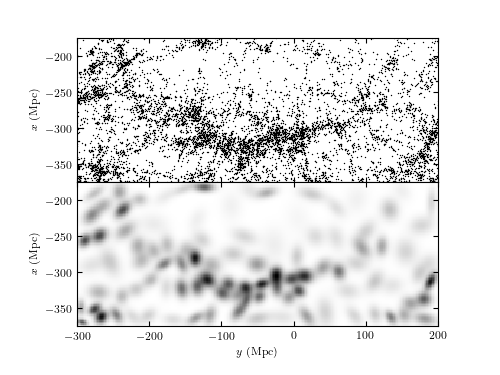# Mixture Model of SDSS Great Wall¶

Figure 6.7

A two-dimensional mixture of 100 Gaussians (bottom) used to estimate the number density distribution of galaxies within the SDSS Great Wall (top). Compare to figures 6.3 and 6.4, where the density for the same distribution is computed using both kernel density and nearest-neighbor-based estimates.@pickle_results: computing results and saving to 'great_wall_GMM.pkl'
converged: True

# Author: Jake VanderPlas
#   The figure produced by this code is published in the textbook
#   "Statistics, Data Mining, and Machine Learning in Astronomy" (2013)
#   To report a bug or issue, use the following forum:

from __future__ import print_function

import numpy as np
from matplotlib import pyplot as plt

from sklearn.mixture import GaussianMixture
from astroML.datasets import fetch_great_wall
from astroML.utils.decorators import pickle_results

#----------------------------------------------------------------------
# This function adjusts matplotlib settings for a uniform feel in the textbook.
# Note that with usetex=True, fonts are rendered with LaTeX.  This may
# result in an error if LaTeX is not installed on your system.  In that case,
# you can set usetex to False.
if "setup_text_plots" not in globals():
from astroML.plotting import setup_text_plots
setup_text_plots(fontsize=8, usetex=True)

#------------------------------------------------------------
X = fetch_great_wall()

#------------------------------------------------------------
# Create a function which will save the results to a pickle file
#  for large number of clusters, computation will take a long time!
@pickle_results('great_wall_GMM.pkl')
def compute_GMM(n_clusters, max_iter=1000, tol=3, covariance_type='full'):
clf = GaussianMixture(n_clusters, covariance_type=covariance_type,
max_iter=max_iter, tol=tol, random_state=0)
clf.fit(X)
print("converged:", clf.converged_)
return clf

#------------------------------------------------------------
# Compute a grid on which to evaluate the result
Nx = 100
Ny = 250
xmin, xmax = (-375, -175)
ymin, ymax = (-300, 200)

Xgrid = np.vstack(map(np.ravel, np.meshgrid(np.linspace(xmin, xmax, Nx),
np.linspace(ymin, ymax, Ny)))).T

#------------------------------------------------------------
# Compute the results
#
# we'll use 100 clusters.  In practice, one should cross-validate
# with AIC and BIC to settle on the correct number of clusters.
clf = compute_GMM(n_clusters=100)
log_dens = clf.score_samples(Xgrid).reshape(Ny, Nx)

#------------------------------------------------------------
# Plot the results
fig = plt.figure(figsize=(5, 3.75))

ax.scatter(X[:, 1], X[:, 0], s=1, lw=0, c='k')

ax.set_xlim(ymin, ymax)
ax.set_ylim(xmin, xmax)

ax.xaxis.set_major_formatter(plt.NullFormatter())
plt.ylabel(r'$x\ {\rm (Mpc)}$')

ax.set_xlabel(r'$y\ {\rm (Mpc)}$')
ax.set_ylabel(r'$x\ {\rm (Mpc)}$')